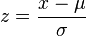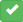# SchoolDigger.com Ranking Methodology

## Summary:

SchoolDigger ranks schools by each school's Average Standard Score. The school with the highest Average Standard Score is ranked #1 in the state.

## Why Standard Scores?

Comparing test scores from different grades and tests is problematic. As an example: from the table below, can you tell which school is performing best in math?

Lincoln Elementary 5th 90.3
Smith Elementary 4th 81.5
Jefferson Elementary 6th 75.0

The truth is, we can't tell from this data. The 4th grade test may be much more difficult than the 5th grade test, and therefore a lower score might actually be the "better" one.

So for a better method in comparing proficiency scores across different grades and tests, we map these raw scores onto a Standard Normal Distribution. This statistical method maps these numbers onto the same shaped bell-curve so that we can compare scores from different grades and tests.

For a terrific explanation of the process and why it is useful, see Standard Normal Distribution at mathisfun.com.

## How we calculate a school's Average Standard Score.

We will now walk through the mathematical steps to calculate a school's Average Standard Score. Let's use data from a fictional school: Smith Elementary in Topeka, Kansas.

In 4th grade math, Smith Elementary's students performed at an 81.5 percent proficiency level.

Math 4th 81.5%

We first calculate a Z-Score for Smith Elementary's 4th grade math score. A Z-Score is the multiple of standard deviations that Smith's 4th grade math score is from the 4th Kansas state mean (average) score. A positive Z-Score means the school score is above the Kansas state mean score, and a negative Z-Score means the score is below the Kansas state mean score.

The Z-Score calculation is:Where χ is the 4th grade math proficiency score, μ is the mean score of all Kansas 4th grade math scores, and σ is the standard deviation of the Kansas 4th grade math scores.

In our case, the mean score for Kansas 4th grade math statewide (μ) is 59.960, and the standard deviation (σ) is 18.018.

So, the calculated Z-Score for Smith Elementary is 1.195:

Test Grade % Proficient State Mean Score State Standard
Deviation
Z-Score
Math 4th 81.5% 59.96 18.02 1.195

This Z-Score is then mapped using the standard normal distribution, which gives us our Standard Score:

Test Grade % Proficient State Mean Score State Standard
Deviation
Z-Score Standard Score
Math 4th 81.5% 59.96 18.02 1.195 87.12%

We do this for all the tests from Smith Elementary and calculate an Average Standard Score:

Test Grade % proficient State Average State Standard
Deviation
Z-Score Standard Score
Math 3rd 85.90 64.38 17.09 1.26 89.60
Math 4th 81.50 61.06 18.06 1.13 87.12
Math 5th 68.90 61.83 17.08 0.41 66.07
Math 6th 73.20 59.85 17.28 0.77 78.00
Reading 3rd 87.20 72.78 14.47 1.00 84.05
Reading 4th 87.30 71.78 14.45 1.07 85.86
Reading 5th 83.60 72.14 14.70 0.78 78.23
Reading 6th 77.50 71.15 14.21 0.45 67.26
Science 5th 78.70 65.72 18.04 0.72 76.42
Writing 4th 77.80 60.85 17.65 0.96 83.16
Average: 79.58

So the final Average Standard Score for Smith Elementary is 79.58%. Our final ranking is based on this score, which is used in the rankings list:

Rank School Average Standard Score
1 Lincoln Elementary 82.83
2 Smith Elementary 79.58
3 Jefferson Elementary 62.14